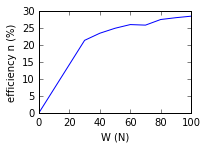# Example 8.1 Simple Machine¶

In :
from __future__ import division
import math
# Initilization of variables
VR=6 # Velocity ratio
P=20 #N # Effort
# Calculations
#(a)
P_actual=P #N
W_actual=W #N
MA=W/P # where, M.A= Mechanical advantage
E=(MA/VR)*100 #% # Where E= efficiency
#(b)
# Now ideal effort required is,
P_ideal=W/VR #N
# Effort loss in friction is, (Le)
Le=P_actual-P_ideal #N # Effort loss in friction
#(c)
W_ideal=P*VR #N
F=W_ideal-W_actual # N
# Results
print('(a) The efficiency of the machine is %f percent'%E)
print('(b) The effort loss in friction of the machine is %f N'%Le)
print('(c) The Frictional load of the machine is %d N'%F)

(a) The efficiency of the machine is 83.333333 percent
(b) The effort loss in friction of the machine is 3.333333 N
(c) The Frictional load of the machine is 20 N


# Example 8.2 Simple machines performance¶

In :
from __future__ import division
import math
%matplotlib inline
from matplotlib.pyplot import plot,subplot,xlabel,ylabel,text,show
import math
import numpy as np

# Initilization of variables
V_r=20 # Velocity ratio
# Values from the table # Variables have been assumed
# Values of W in N
W=[30,40,50,60,70,80,90,100]
# P in N
P=[7,8.5,10,11.5,13.5,14.5,16,17.5]
MA=[]
MA[:]=[W[i]/P[i] for i in range(0,8)]
# Efficiency (n)
n = np.asarray(MA) * (V_r** -1) * 100
# Calculations
# Part (a)- Realtionship between W & P
# Here part a cannot be solved as it has variables which cannot be defined in Scilab. Ref.textbook for the solution
# Part (b)- Graph between W & efficiency n(eta)
x=[0,W,W,W,W,W,W,W,W] # values for W # N
y=[0,n,n,n,n,n,n,n,n] # values for efficiency n (eta) # %
subplot(221)
xlabel("W (N)")
ylabel("efficiency n (%)")
plot(x,y)
# Results
print('The graph is the solution')
# The value of m is found by drawing a straight line in the graph and by taking its slope
# The curve of the graph may differ from textbook because of the graphical calculation.

The graph is the solution# Example 8.3 Performance of Machine¶

In :
from __future__ import division
import math
# Calculations
P_actual=100 #N # Effort
n=4 # no of pulleys
# Calculations
# for 1st system of pulleys having 4 movable pulleys, Velocity ratio is
VR=2**(n) # Velocity Ratio
# If the machine were to be ideal(frictionless)
MA=VR # Here, M.A= mechanical advantage
# For a load of 1360 N, ideal effort required is
P_ideal=W_actual/VR #N
# Effort loss in friction is,
P_friction=P_actual-P_ideal #N
# For a effort of 100 N, ideal load lifted is,
W_ideal=VR*100 #N
# Load lost in friction is,
W_friction=W_ideal-W_actual # N
# Results
print('(a) The effort wasted in friction is %d N'%P_friction)
print('(b) The load wasted in friction is %d N'%W_friction)

(a) The effort wasted in friction is 15 N
(b) The load wasted in friction is 240 N


# Example 8.4 Performance of a machine¶

In :
from __future__ import division
import math
# Initilization of variables
W=1000 #N # Load to be lifted
n=5 # no. of pulleys
E=75 #% # Efficiency
# Calculations
# Velocity Ratio is given as,
VR=n
MA=(E/100)*VR # from formulae, Efficiency=E=M.A/V.R
P=W/MA #N # Effort required
# Results
print('The effort required to lift the load of 1000 N is %f N'%P)

The effort required to lift the load of 1000 N is 266.666667 N


# Example 8.5 Simple screw jack¶

In :
from __future__ import division
import math
# Initilization of variables
W=2000 #N # Load to be raised
l=0.70 #m # length of the handle
d=0.05 #m # diameter of the screw
p=0.01 #m # pitch of the screw
mu=0.15 # coefficient of friction at the screw thread
E=1 # efficiency
# Calculations
phi=math.degrees(math.atan(mu)) #degree
theta=math.degrees(math.atan(p/(math.pi*d))) #degree # where theta is the Helix angle
# Force required at the circumference of the screw is,
P=W*(math.tan((theta+phi)*math.pi/180)) # N #
# Force required at the end of the handle is,
F=(P*(d/2))/l #N
# Force required (Ideal case)
VR=2*math.pi*l/p
MA=E*VR # from formulae E=M.A/V,R
P_ideal=W/MA #N # From formulae, M.A=W/P
# Results
print('The force required at the end of the handle is %f N'%F)
print('The force required if the screw jack is considered to be an ideal machine is %f N'%P_ideal)

The force required at the end of the handle is 15.408712 N
The force required if the screw jack is considered to be an ideal machine is 4.547284 N